##### Quantum Physics For Dummies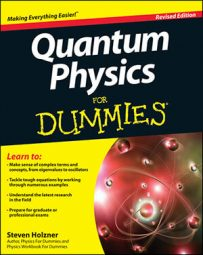At some point, your quantum physics instructor may want you to find the total energy equation for three-dimensional free particle problems. The total energy of the free particle is the sum of the energy in three dimensions:

E = Ex + Ey + Ez

With a free particle, the energy of the x component of the wave function is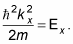And this equation works the same way for the y and z components, so here’s the total energy of the particle: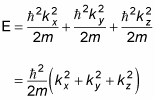Note that kx2 + ky2 + kz2 is the square of the magnitude of k — that is,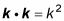Therefore, you can write the equation for the total energy as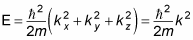Note that because E is a constant, no matter where the particle is pointed, all the eigenfunctions of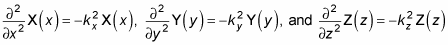are infinitely degenerate as you vary kx, ky, and kz.